# A bent bar is supported with a fixed end at A. There are two forces applied...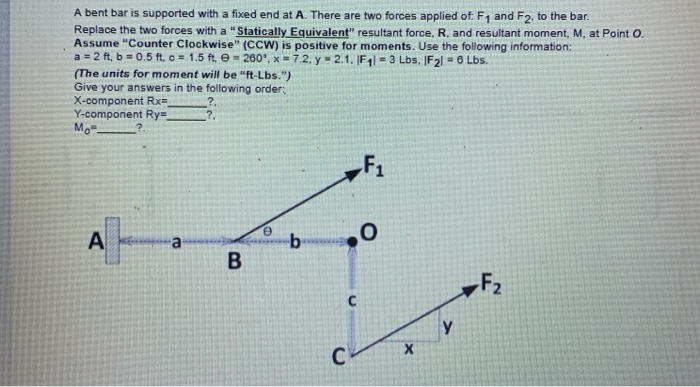A bent bar is supported with a fixed end at A. There are two forces applied of F1 and F2, to the bar. Replace the two forces with a "Statically Equivalent" resultant force, R. and resultant moment, M, at Point O. Assume "Counter Clockwise" (CCW) is positive for moments. Use the following information: a = 2t, b = 0.5 ft. c = 1.5 ft - 260'. * - 7.2. y = 2.1. F11 = 3 Lbs. IF21 - Lbs. (The units for moment will be "ft-Lbs.") Give your answers in the following orders X-component Rx ? Y-component Ry M.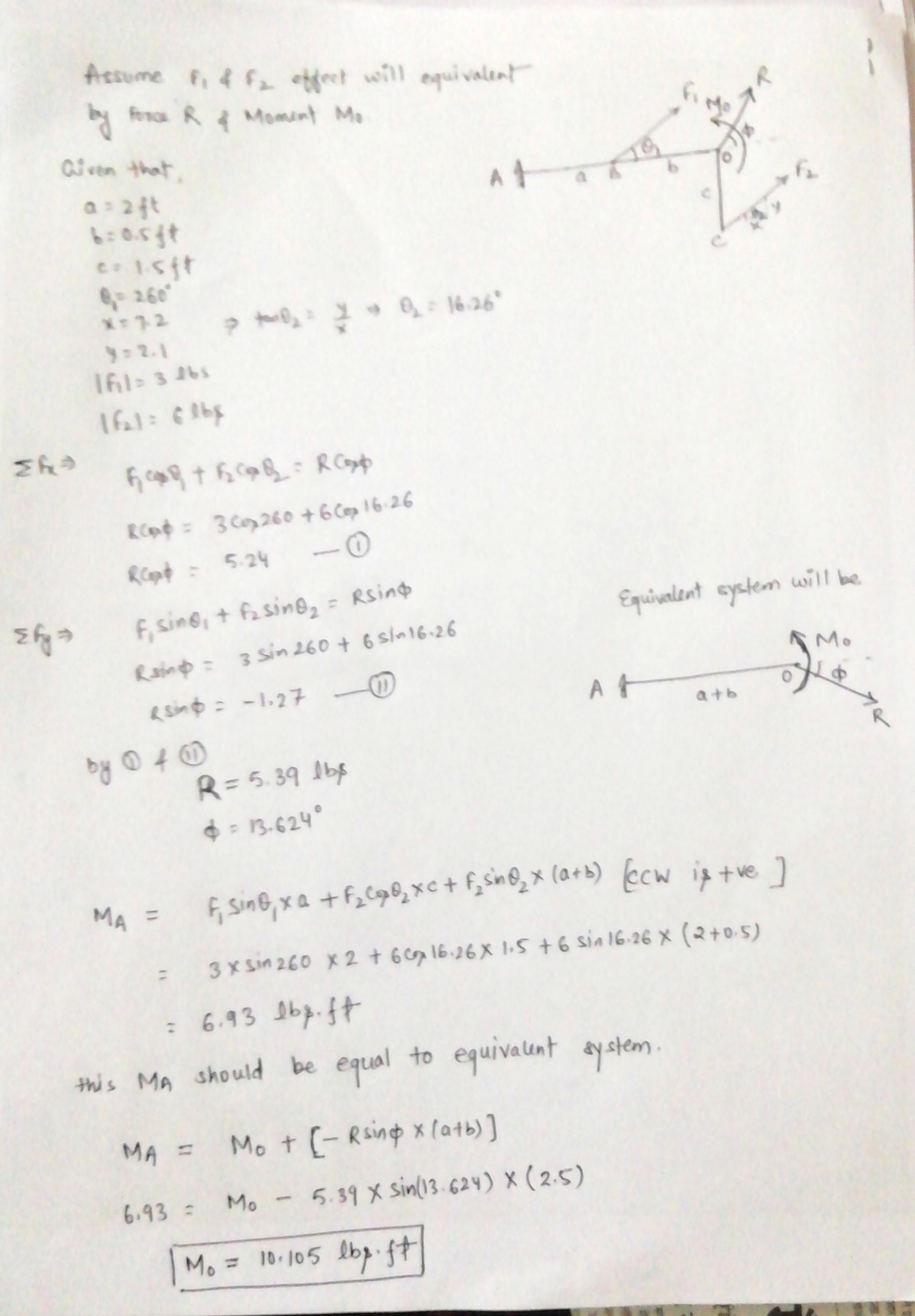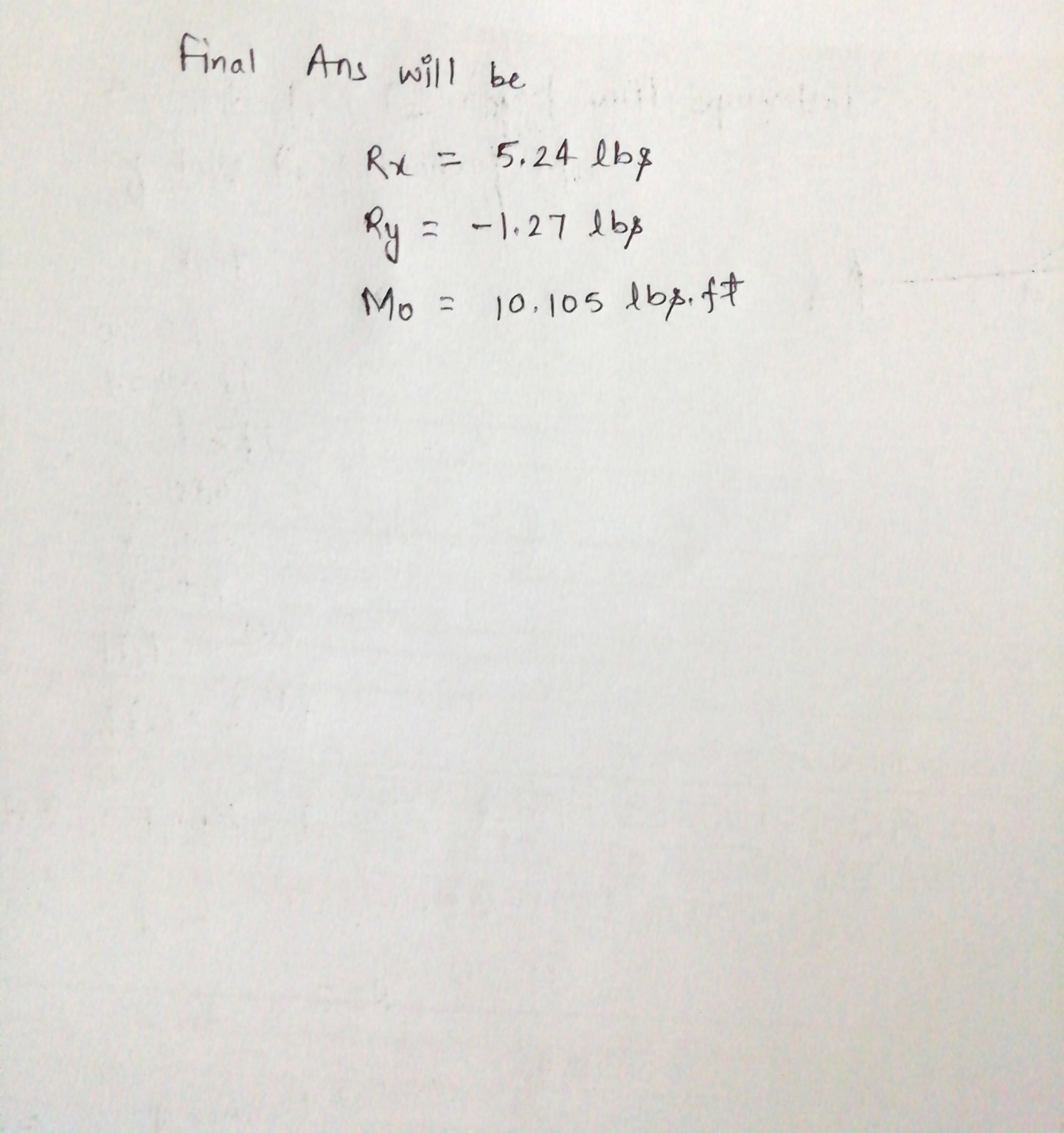PLEASE RATE THE ANSWER

THANKS

#### Earn Coin

Coins can be redeemed for fabulous gifts.

Similar Homework Help Questions
• ### The bent rod is supported at A, B, and C by smooth journal bearings. A)Compute the...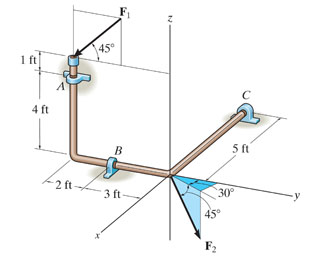The bent rod is supported at A, B, and C by smooth journal bearings. A)Compute the x, y, z components of reaction at the bearing A if the rod is subjected to forces F1 = 500 lb and F2 = 250 lb.F1 lies in the y?z plane. The bearings are in proper alignment and exert only force reactions on the rod. B)Compute the x, y, z components of reaction at the bearing B C)Compute the x, y, z components of...

• ### Two forces, F1 and F2, act at a point, as shown in the picture. (Figure 1)...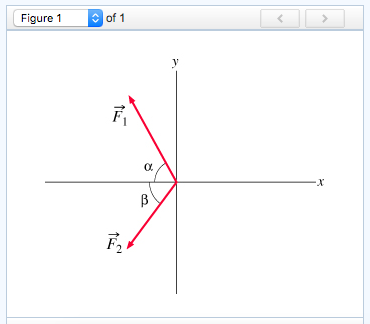Two forces, F1 and F2, act at a point, as shown in the picture. (Figure 1) F1 has a magnitude of 8.40 N and is directed at an angle of a = 59.0 ? above the negative xaxis in the second quadrant. F2 has a magnitude of 6.40 N and is directed at an angle of b = 54.1 ? below the negative x axis in the third quadrant. a) What is the x component Fx of the resultant force?  Express...

• ### (2) jahë From fig (1) Determine (a) the result (component of forces in x-axis (Rx F3...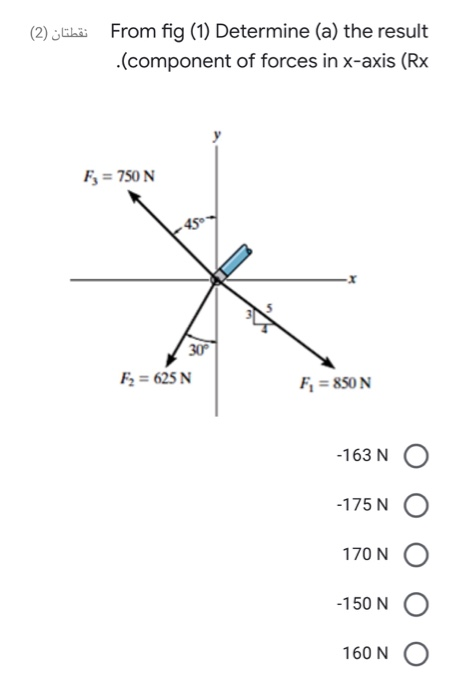(2) jahë From fig (1) Determine (a) the result (component of forces in x-axis (Rx F3 = 750 N 30 F2 = 625 N F, = 850 N -163 N. -175 N O 170 N -150 N 160 N (2) jlbä b) The result component of forces) .(in y-axis (Ry -545 N -478 N -485 N 550 N -521 N نقطتان (2) c) The magnitude and of the) resultant force 610 N O 546 N 605 N 521 N O...

• ### Two forces, F? 1 and F? 2, act at a point, as shown in the picture....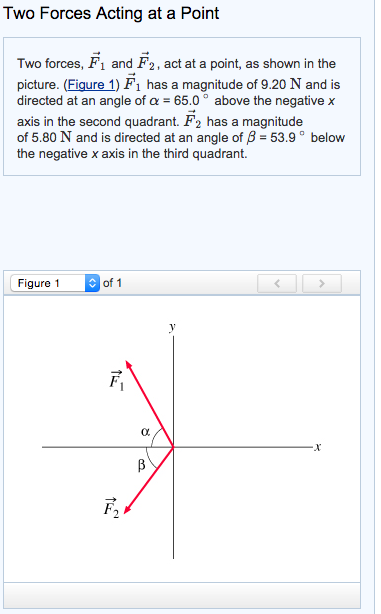Two forces, F? 1 and F? 2, act at a point, as shown in the picture. (Figure 1) F? 1 has a magnitude of 9.20 N and is directed at an angle of ? = 65.0 ? above the negative xaxis in the second quadrant. F? 2 has a magnitudeof 5.80 N and is directed at an angle of ? = 53.9 ? below the negative x axis in the third quadrant. Part A What is the x component Fx...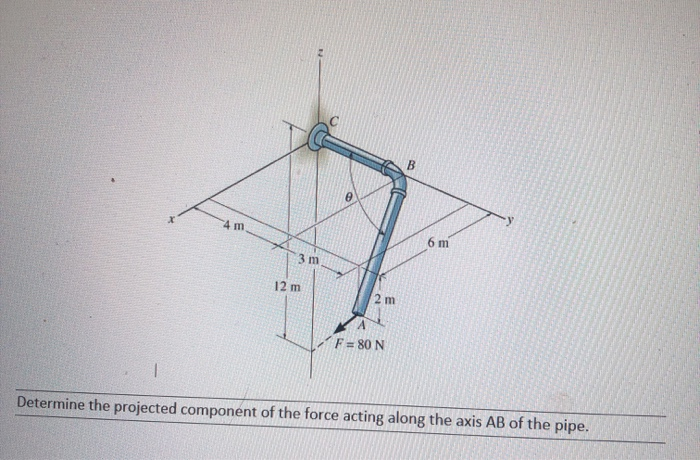please help solve for what is asked in orobkems A-H and the free body diagrams as well. Will upvote if correct A B C D E F G H B 4 m 6 m 3 m 12 m 2 m F=80 N Determine the projected component of the force acting along the axis AB of the pipe. 60° A The 30kg pipe is supported at A by a system of five cords. Determine the force in each cord for equilibrium....